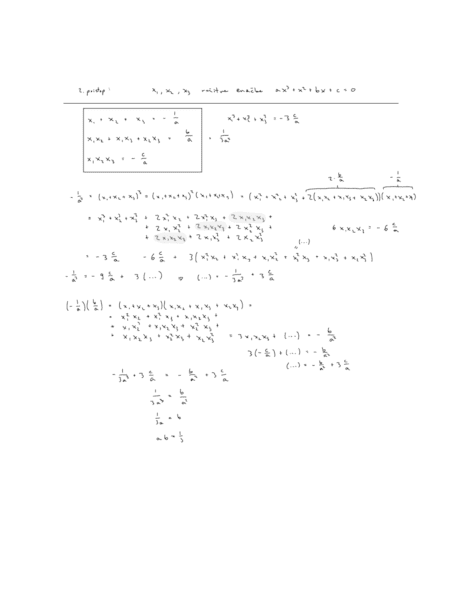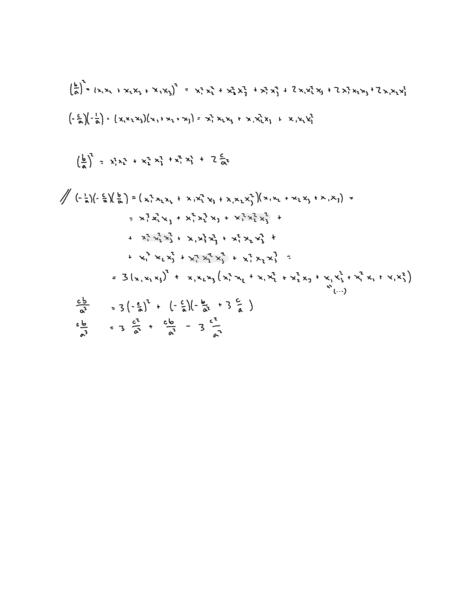# Finding the coefficients of a polynomial given some restriction

## Homework Statement

Find all ##a,b,c\in\mathbb{R}## for which the zeros of the polynomial ##az^3+z^2+bz+c=0## are in this relation $$z_1^3+z_2^3+z_3^3=3z_1z_2z_3$$

## Homework Equations

we know that if we have a polynomial of degree 3 the zeroes have relation in this case
##z_1+z_2+z_3=-1/a##
##z_1z_2+z_1z_3+z_2z_3=b/a ##
##z_1z_2z_3=-c/a##

## The Attempt at a Solution

I've tried doing something with the vietto rules but I have not really gotten anything. Any tip on how to even start the problem would be really appreciated#### Attachments

fresh_42
Mentor
2021 Award
There is another symmetry. Say ##z_1\in \mathbb{R}##, then ##z_{2,3}=x \pm iy##.

Mark44
Mentor
The images in post #1 are unreadable, at least by me.

Ray Vickson
Homework Helper
Dearly Missed

## Homework Statement

Find all ##a,b,c\in\mathbb{R}## for which the zeros of the polynomial ##az^3+z^2+bz+c=0## are in this relation $$z_1^3+z_2^3+z_3^3=3z_1z_2z_3$$

## Homework Equations

we know that if we have a polynomial of degree 3 the zeroes have relation in this case
##z_1+z_2+z_3=-1/a##
##z_1z_2+z_1z_3+z_2z_3=b/a ##
##z_1z_2z_3=-c/a##

## The Attempt at a Solution

I've tried doing something with the vietto rules but I have not really gotten anything. Any tip on how to even start the problem would be really appreciated
View attachment 232485 View attachment 232486

If ##z_i## is a root then $$z_i^3 = -\frac{c}{a} - \frac{b}{a} z_i - \frac{1}{a} z_i^2,$$
and we can find ##\sum z_i^2## from the expansion of ##(z_1+z_2+z_3)^2.##